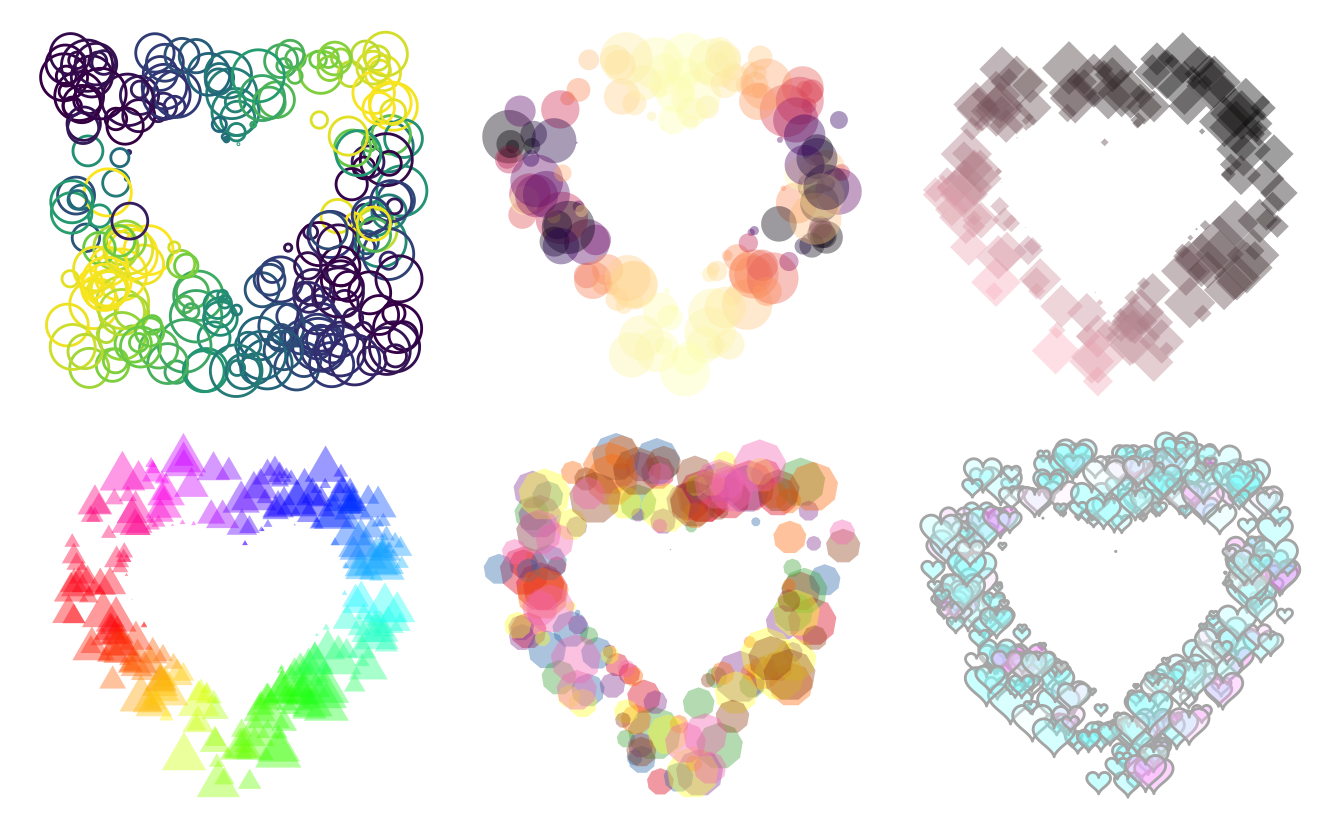# Why are we here?

Some days ago I say this little cute pen and it sparked something inside me.

See the Pen Heart is Home by Chris Gannon (@chrisgannon) on CodePen.

I throw together some lines of code and took my first splash into using simple Features. This post is not meant as an introduction to sf, a great introduction to the sf objects is made by Jesse Sadler.

``````library(tidyverse)
library(sf)
library(patchwork)``````

# First run

First we create the center shape. I have gone for heart shape, for which I found a parametric expression, I have wrapped all of this in a little function such that I can specify the number of points the polygon has.

``````heart_fun <- function(n) {
t <- c(seq(0, 2 * pi, length.out = n), 0)

out <- data.frame(
x = c(16 * sin(t) ^ 3),
y = 13 * cos(t) - 5 * cos(2 * t) - 2 * cos(3 * t) - cos(4 * t)
)
out <- as.matrix(out)
out <- list(out)
st_polygon(out)
}``````

Lets check that the function works

``````heart_fun(100)
## POLYGON ((0 5, 0.004082058 5.082247, 0.03245962 5.325084, 0.1084517 5.716992, 0.2534598 6.239393, 0.4860975 6.867539, 0.8214215 7.571701, 1.270293 8.31857, 1.838891 9.072817, 2.528404 9.798711, 3.334892 10.46172, 4.24935 11.03003, 5.25795 11.47583, 6.342465 11.77642, 7.480851 11.915, 8.647981 11.88112, 9.816481 11.67082, 10.95766 11.28641, 12.04251 10.736, 13.04268 10.03268, 13.93146 9.193568, 14.68474 8.238708, 15.28179 7.189845, 15.70606 6.069255, 15.94569 4.898625, 15.99396 3.698075, 15.8495 2.485356, 15.51639 1.275288, 15.00393 0.07943237, 14.32642 -1.093982, 13.50257 -2.239884, 12.5549 -3.355982, 11.50893 -4.442201, 10.3923 -5.5, 9.233833 -6.531618, 8.062492 -7.539309, 6.906432 -8.524629, 5.792014 -9.487815, 4.742924 -10.42731, 3.77938 -11.33948, 2.917472 -12.21848, 2.168659 -13.05638, 1.539432 -13.84345, 1.031163 -14.56857, 0.6401401 -15.21987, 0.3577924 -15.78537, 0.1710904 -16.25367, 0.06311066 -16.61466, 0.01374229 -16.86016, 0.0005110288 -16.9844, -0.0005110288 -16.9844, -0.01374229 -16.86016, -0.06311066 -16.61466, -0.1710904 -16.25367, -0.3577924 -15.78537, -0.6401401 -15.21987, -1.031163 -14.56857, -1.539432 -13.84345, -2.168659 -13.05638, -2.917472 -12.21848, -3.77938 -11.33948, -4.742924 -10.42731, -5.792014 -9.487815, -6.906432 -8.524629, -8.062492 -7.539309, -9.233833 -6.531618, -10.3923 -5.5, -11.50893 -4.442201, -12.5549 -3.355982, -13.50257 -2.239884, -14.32642 -1.093982, -15.00393 0.07943237, -15.51639 1.275288, -15.8495 2.485356, -15.99396 3.698075, -15.94569 4.898625, -15.70606 6.069255, -15.28179 7.189845, -14.68474 8.238708, -13.93146 9.193568, -13.04268 10.03268, -12.04251 10.736, -10.95766 11.28641, -9.816481 11.67082, -8.647981 11.88112, -7.480851 11.915, -6.342465 11.77642, -5.25795 11.47583, -4.24935 11.03003, -3.334892 10.46172, -2.528404 9.798711, -1.838891 9.072817, -1.270293 8.31857, -0.8214215 7.571701, -0.4860975 6.867539, -0.2534598 6.239393, -0.1084517 5.716992, -0.03245962 5.325084, -0.004082058 5.082247, -2.350945e-46 5, 0 5))``````

and that it plots correctly.

``plot(heart_fun(100))``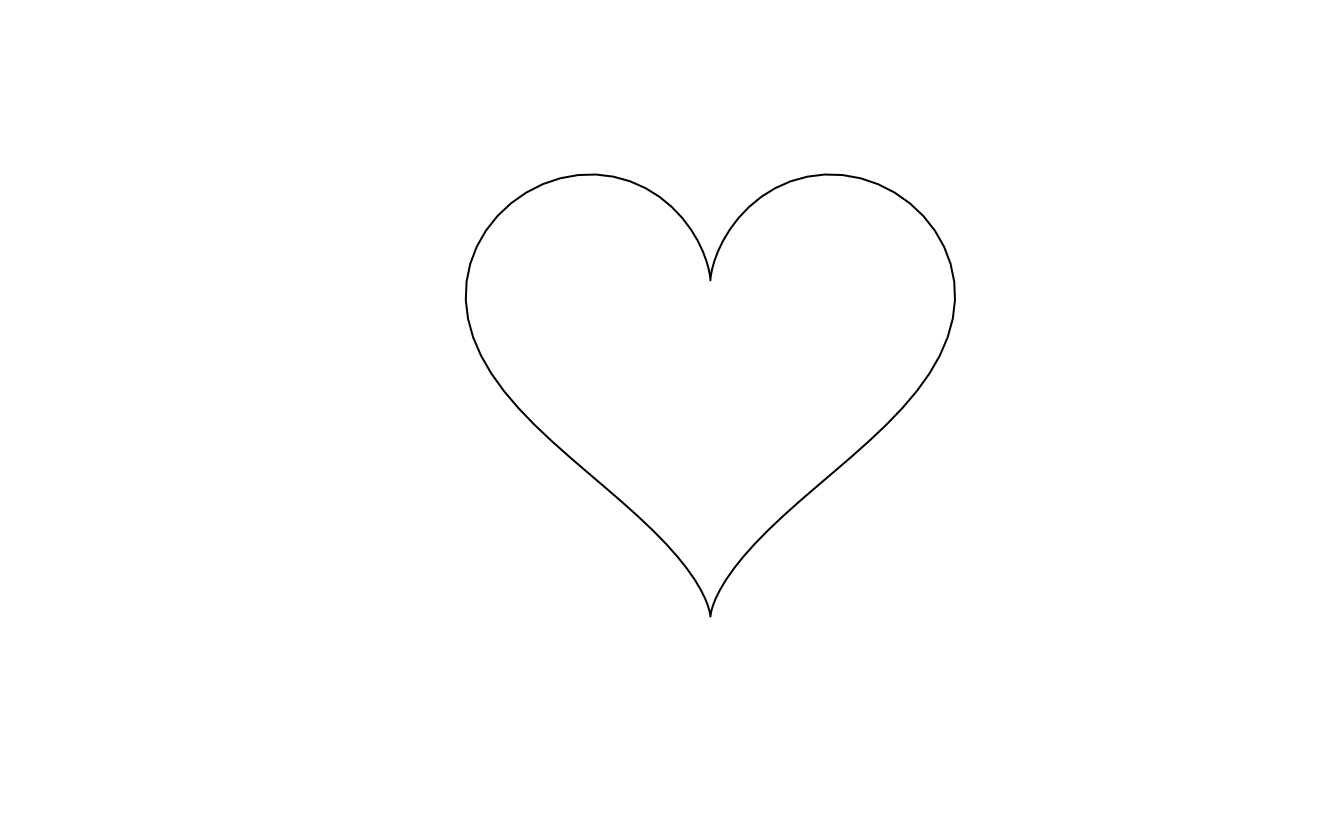We also create a helper function to create a unit circle.

``````circle_fun <- function(n) {
t <- c(seq(0, 2 * pi, length.out = n), 0)

out <- data.frame(
x = sin(t),
y = cos(t)
)
out <- as.matrix(out)
out <- list(out)
st_polygon(out)
}

plot(circle_fun(100))``````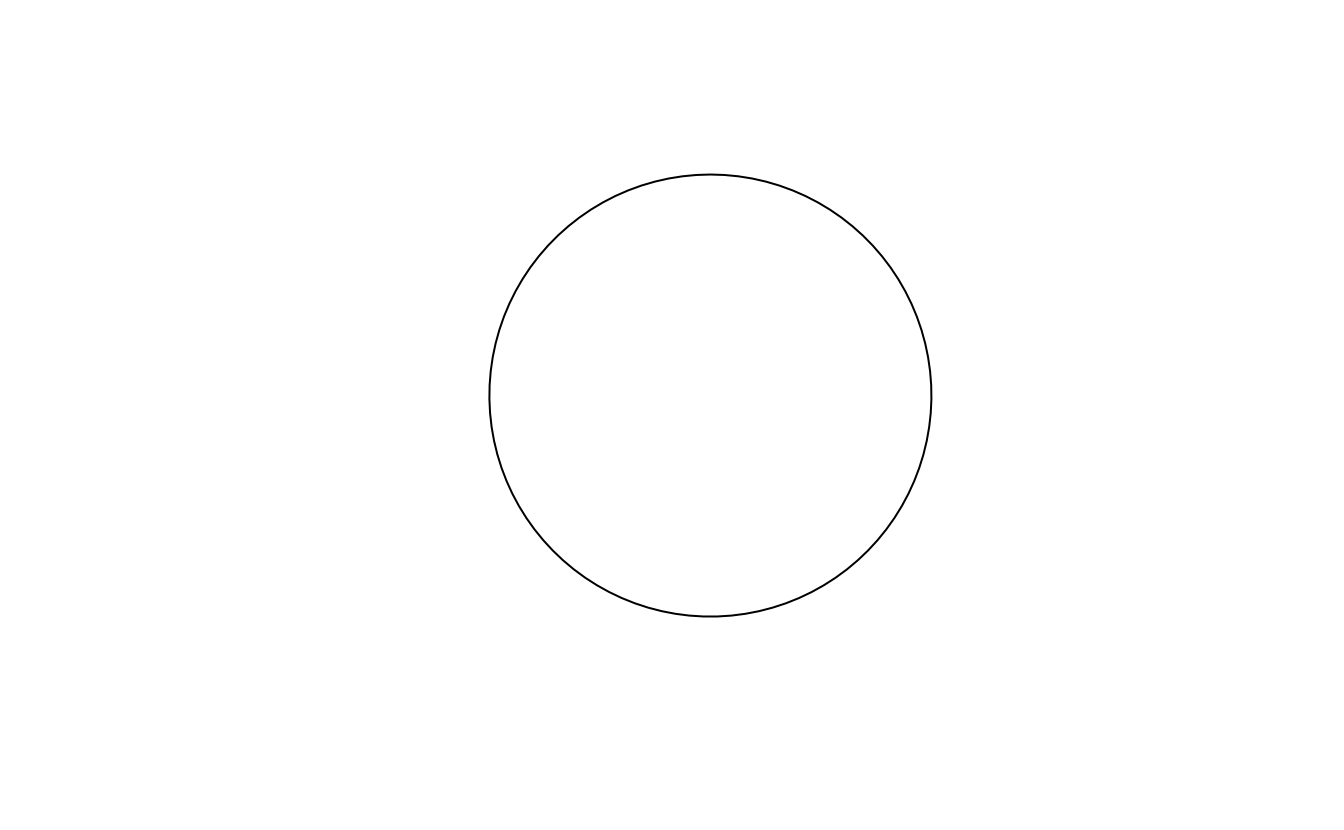So we have a heart shape, lets check the boundaries of that shape.

``````st_bbox(heart_fun(100))
##      xmin      ymin      xmax      ymax
## -15.99396 -16.98440  15.99396  11.91500``````

Lets generate a sf polygon of both the heart and circle polygon.

``````circle <- circle_fun(100)
heart <- heart_fun(100)``````

Next we want to generate a list of candidate points where we try to place circles. for now we will just randomly sample between -25 and 25 on the x axis and -20 and 20 on the y axis. then we will save them as a sf object.

``````points <- data.frame(x = runif(250, -25, 25),
y = runif(250, -20, 20)) %>%
sf::st_as_sf(coords = c(1, 2))

plot(points)``````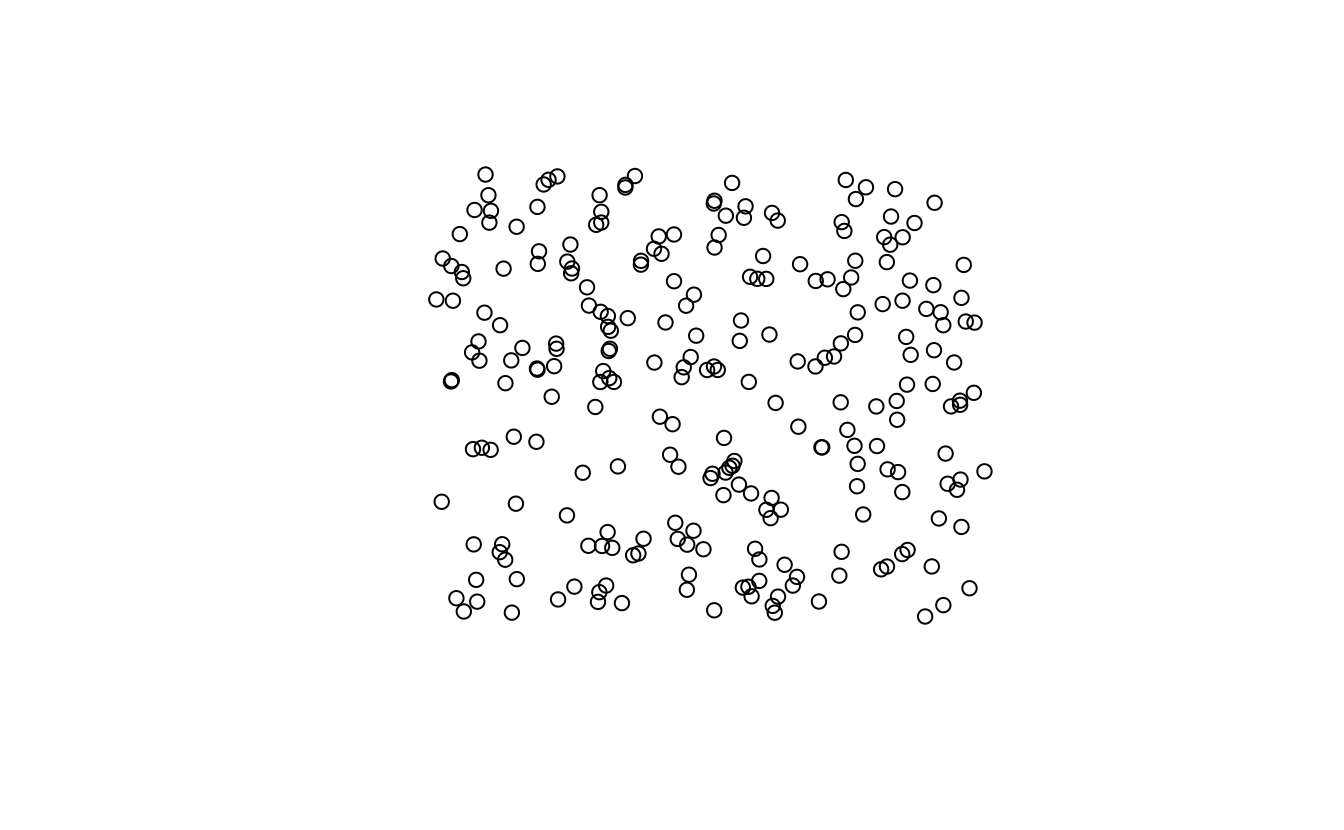Next we will filter the points such that we only consider points that are outside the heart shape.

``````points <- points[!lengths(st_intersects(points, heart)), ]
plot(points)``````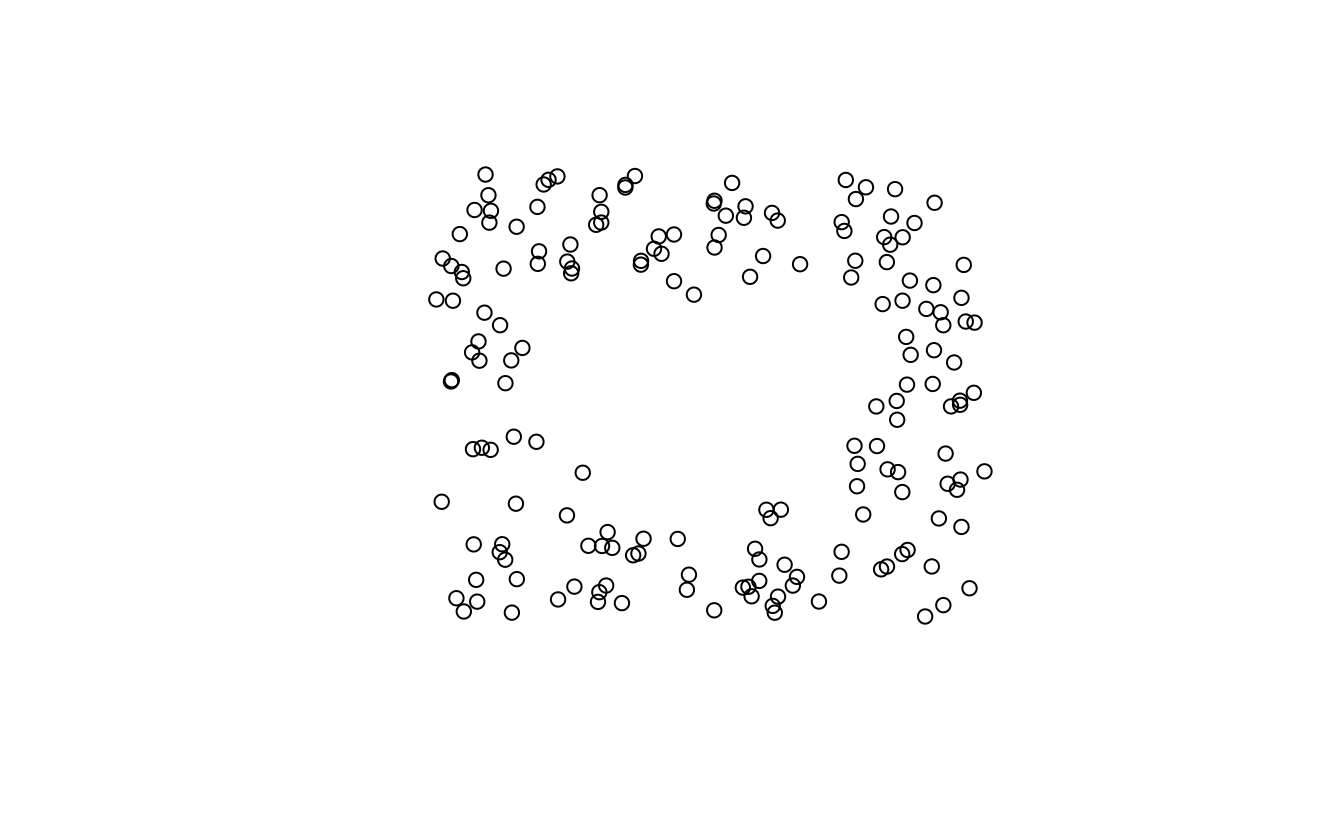Next we will loop through every single point and calculate the distance (using `st_distance`) from the point to the heart. then we will place a circle on that point and scale it such that is has a radius equal to the distance we calculated. That way the heart shape should show given enough points.

``````all_polygons <- map(points[],
~ (circle * st_distance(heart, .x, by_element = TRUE)) + .x) %>%
st_sfc()``````
``plot(all_polygons)``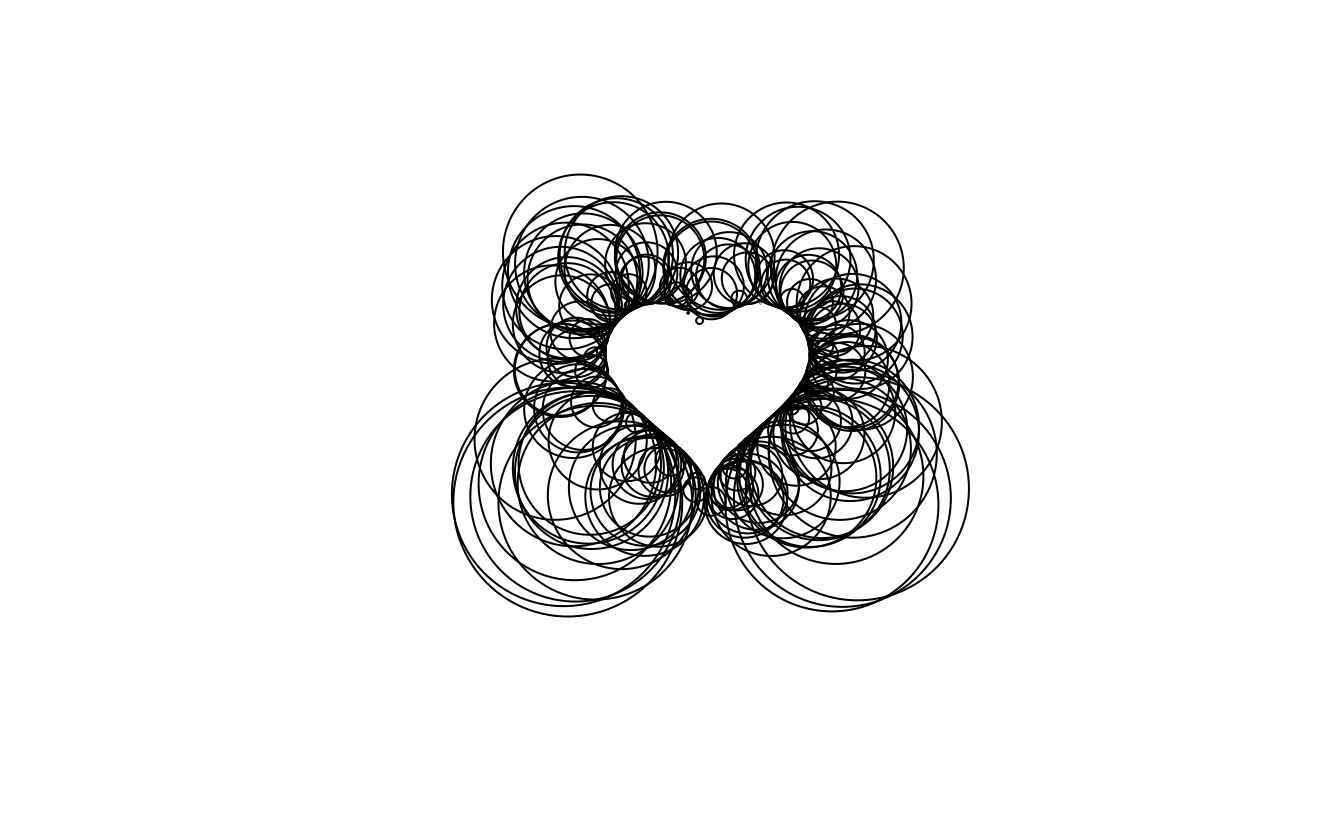And we get something nice! however some of the circle become quite big. Lets bound the radius and give it some variation.

``````bound <- function(x, limit) {
ifelse(x > limit, runif(1, limit / 4, limit), x)
}

all_polygons <- map(points[],
~ (circle * bound(st_distance(heart, .x, by_element = TRUE), 4)) + .x) %>%
st_sfc()

plot(all_polygons)``````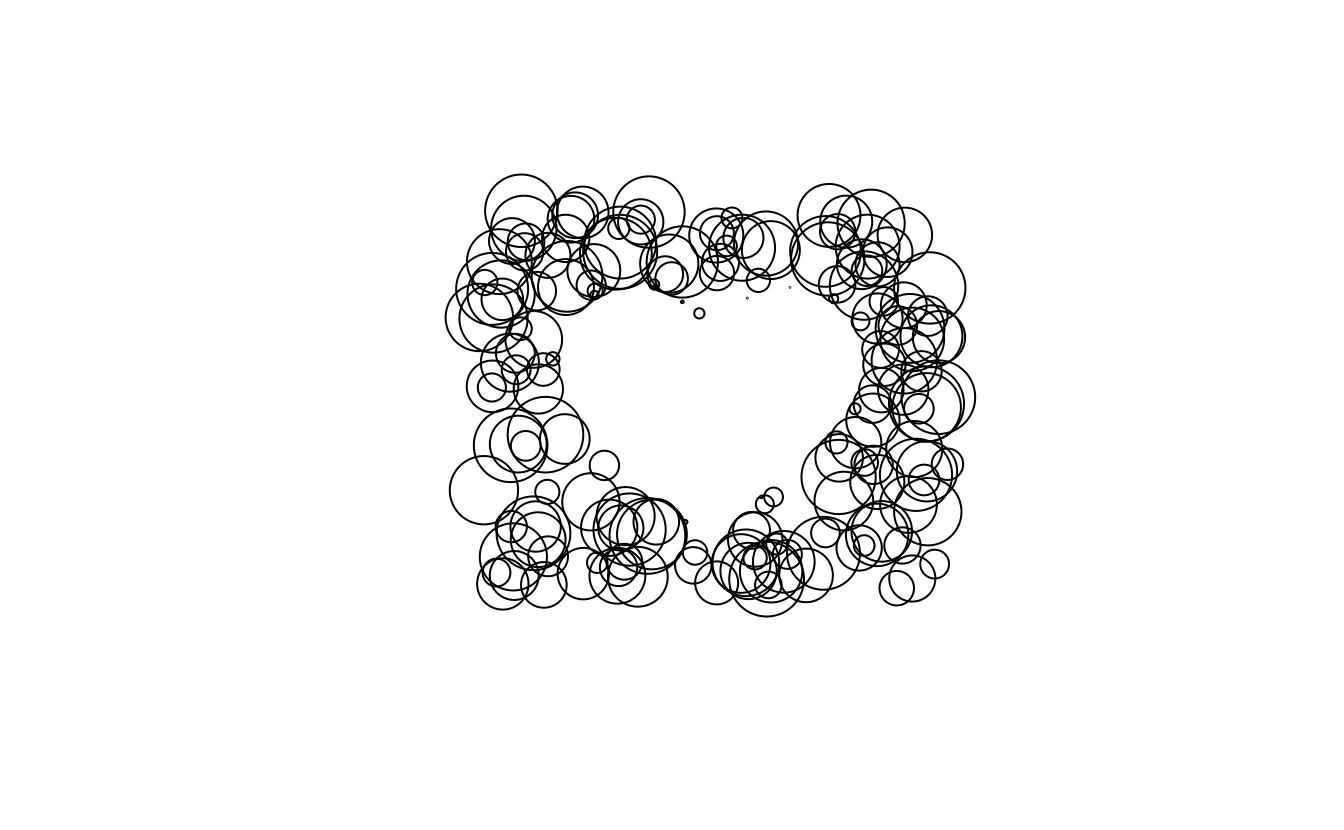Now lets turn this into a data.frame and extract the x and y coordinate so we can use them for coloring.

``````plotting_data <- data.frame(all_polygons) %>%
mutate(x = map_dbl(geometry, ~st_centroid(.x)[]),
y = map_dbl(geometry, ~st_centroid(.x)[])) ``````

Now that we have everything we need we will turn to `ggplot2` to pretty everything up.

``````plotting_data %>%
ggplot() +
geom_sf(aes(color = y, geometry = geometry), alpha = 0.2, fill = NA) +
coord_sf(datum = NA) +
theme_void() +
guides(color = "none")``````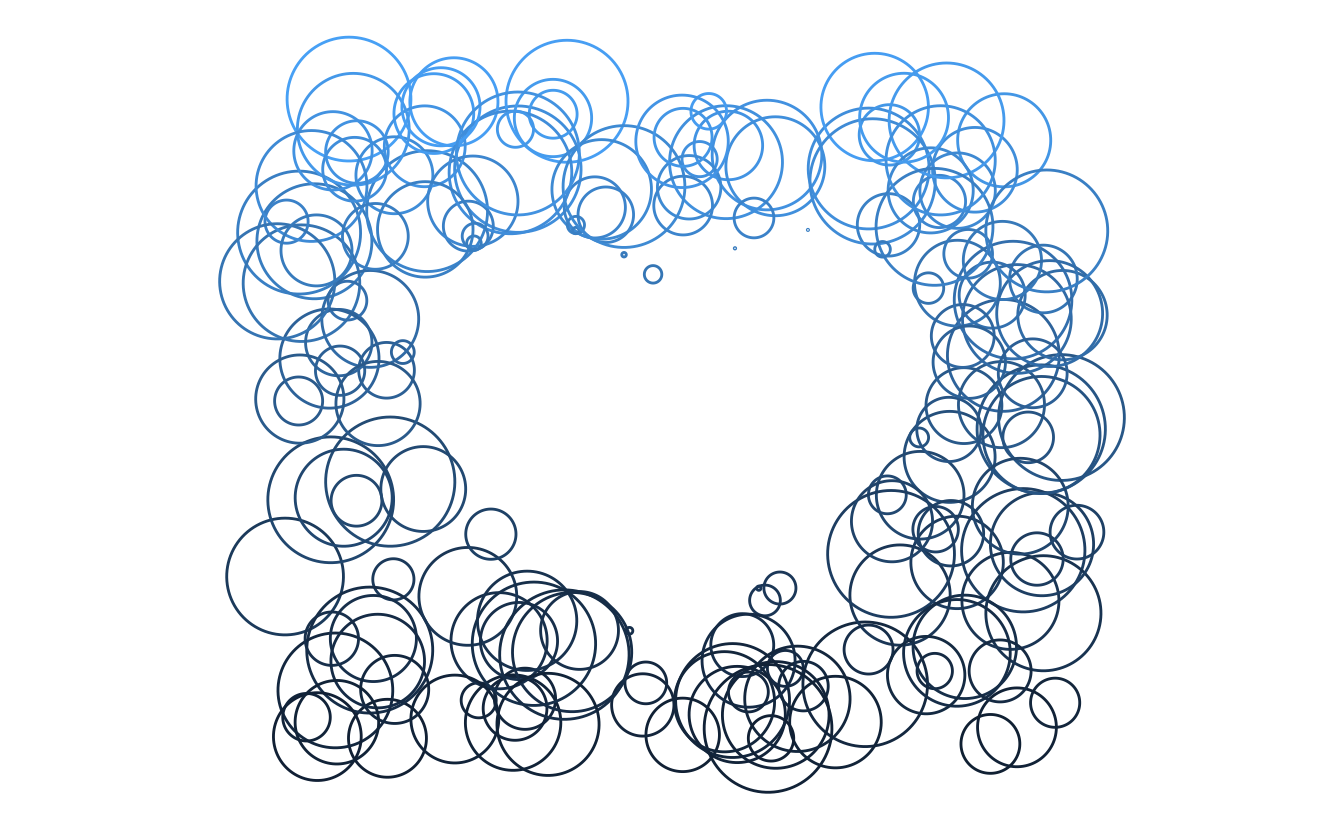And we are done! It looks nice and pretty, now there is a bunch of things we can change.

• color scales
• coloring patterns
• circle arrangement (rectangle, circle, buffer)

# One function plotting

Everything from before is not wrapper up nice and tight in this function.

``````circle_heart <- function(n, center_sf, outside_sf, outside_filter = "None", plotting_margin = 5, ...) {

bound <- function(x, limit) {
ifelse(x > limit, runif(1, limit / 4, limit), x)
}

range <- st_bbox(center_sf)
points <- data.frame(x = runif(n, range[["xmin"]] - plotting_margin,
range[["xmax"]] + plotting_margin),
y = runif(n, range[["ymin"]] - plotting_margin,
range[["ymax"]] + plotting_margin)) %>%
sf::st_as_sf(coords = c(1, 2))

if (outside_filter == "buffer") {
points <- st_intersection(points, st_buffer(center_sf, plotting_margin))
}

points <- points[!lengths(st_intersects(points, center_sf)), ]

all_polygons <- map(points[],
~ (outside_sf * bound(st_distance(center_sf, .x, by_element = TRUE), 4)) + .x) %>%
st_sfc()

plotting_data <- data.frame(all_polygons) %>%
mutate(x = map_dbl(geometry, ~st_centroid(.x)[]),
y = map_dbl(geometry, ~st_centroid(.x)[]))

plotting_data %>%
ggplot() +
geom_sf(..., mapping = aes(geometry = geometry)) +
coord_sf(datum = NA) +
theme_void()
}``````

It returns a simple ggplot2 object that we then can further modify to our visual liking.

``circle_heart(300, heart_fun(100), circle_fun(100))``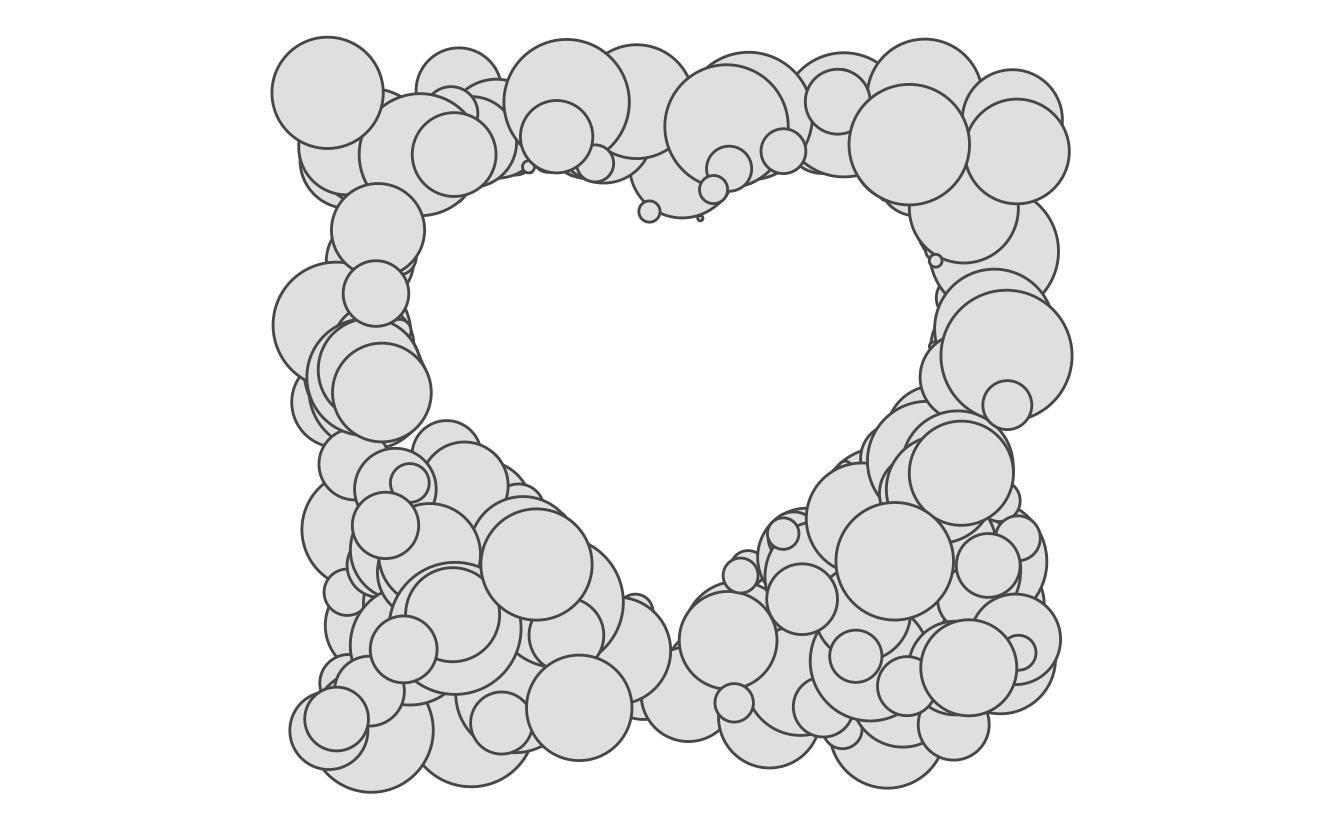A handful of examples

``````p1 <- circle_heart(300, heart_fun(100), circle_fun(100),
plotting_margin = 10, fill = NA) +
aes(color = sin(x / y)) +
scale_color_viridis_c() +
guides(color = "none")

p2 <- circle_heart(300, heart_fun(100), circle_fun(100),
outside_filter = "buffer", plotting_margin = 10, color = NA, alpha = 0.4) +
aes(fill = cos(x / y)) +
scale_fill_viridis_c(option = "A") +
guides(fill = "none")

p3 <- circle_heart(300, heart_fun(100), circle_fun(5),
outside_filter = "buffer", plotting_margin = 10, color = NA, alpha = 0.4) +
aes(fill = x + y) +
scale_fill_gradient(low = "pink", high = "black") +
guides(fill = "none")

p4 <- circle_heart(500, heart_fun(100), circle_fun(4),
outside_filter = "buffer", plotting_margin = 10, color = NA, alpha = 0.4) +
aes(fill = atan2(y, x)) +
guides(fill = "none")

p5 <- circle_heart(300, heart_fun(100), circle_fun(10),
outside_filter = "buffer", plotting_margin = 10, color = NA, alpha = 0.4) +
aes(fill = factor(floor(x * y) %% 8)) +
scale_fill_brewer(palette = "Set1") +
guides(fill = "none")

p6 <- circle_heart(500, heart_fun(100), heart_fun(100) / 20,
outside_filter = "buffer", plotting_margin = 10, color = "grey70", alpha = 0.4) +
aes(fill = (y %% 4) * (x %% 1)) +